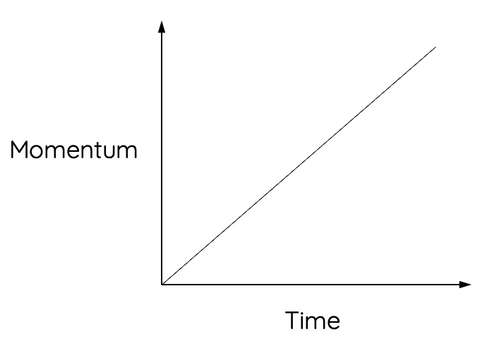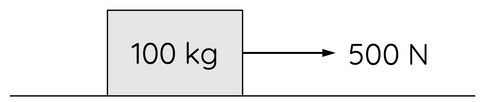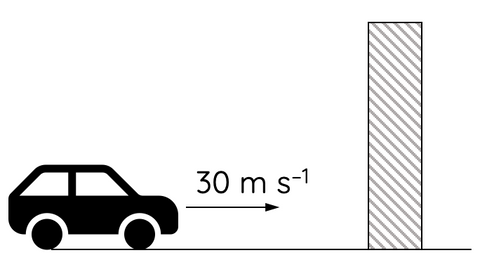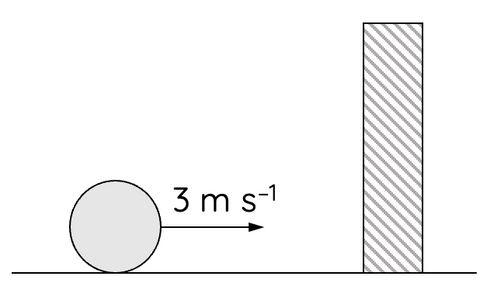# Force, Momentum and Impulse

This topic is part of the HSC Physics course under the section Momentum, Energy and Simple Systems.

### HSC Physics Syllabus

• evaluate the effects of forces involved in collisions and other interactions, and analyse quantitatively the interactions using the concept of impulse Δp=F_{net}Δt

### Force and Momentum

Force, by Newton's second law, is given by:

$$F = m \times a$$

Acceleration is the rate of change of velocity:

$$a = \frac{\Delta v}{\Delta t}$$

$$F = m \times \frac{\Delta v}{\Delta t}$$

Momentum is mass times velocity:

$$p = m \times v$$

The rate of change of momentum:

$$\frac{\Delta p}{\Delta t} = m \times \frac{\Delta v}{\Delta t}$$

Therefore, force is the rate of change of momentum:

$$F = \frac{\Delta p}{\Delta t}$$

### Momentum vs Time Graph

The gradient of a line on a momentum vs time graph represents the rate of change of momentum. Therefore, the gradient in this case represents force.

###For example, the straight line on the graph above suggests that a constant force acts on the mass.

### What is Impulse?

Impulse (I): Impulse is the change in an object's momentum, and it's equal to the product of force (F) and the time (t) during which it acts. Impulse is also a vector quantity, and its direction is the same as the force.

The equation for impulse is:

$$I = \Delta p$$

From the equation for force in terms of p and t, we can derive another equation for impulse:

$$I = F\Delta t$$

The unit for impulse is the same as momentum (kg m s-1) or the product of units of force and time (N s)

### Example 1

A 100 kg mass at rest is acted upon by a 500 N net force to the right as shown for 2.5 seconds.What is the impulse of the force and the final velocity of the mass?

Solution:

First, we can calculate impulse:

$$I = F \times t$$

$$I = (500)(2.5) = 1250 \, \text{N s to the right}$$

Consider impulse as the change in momentum:

$$I = \Delta p$$

Since the mass was at rest, its initial momentum was zero:

$$I = mv$$

$$1250 = (100)v$$

$$v = 12.5 \, m \, s^{-1}$$

### Example 2

An 800 kg car moving at 30 m s–1 collides with a wall. The collision causes the car to come to an abrupt stop in 0.25 s.What magnitude of force does the wall exert on the car during the collision?

Solution:

Consider the change in momentum (impulse) in terms of force and time:

$$\Delta p = F \times t$$

$$mv - mu = F \times t$$

$$(800)(0) - (800)(30) = F(0.25)$$

$$F = - 9.6 \times 10^4 \, N$$

### Example 3

A 0.5 kg ball moving at 3 m s–1 on a frictionless surface collides with a vertical wall as shown. The wall exerts an average force of 120 N on the ball for a duration of 20 ms.(a) What is the final speed of the ball?

(b) How much mechanical energy was lost during the collision?

Solution to part (a):

Consider the change in momentum as the product of force and time:

$$mv - mu = F \times t$$

Let vectors directed to the right be positive (and left to be negative). When the ball collides with the wall, the wall exerts a force on the ball to the left.

$$(0.5)v - (0.5)(3) = (-120)(20 \times 10^{-3})$$

$$v = -1.8 \, m \, s^{-1}$$

Solution to part (b):

Mechanical energy is given by the sum of a mass' kinetic and potential energy. In this case, there is no changes in potential energy.

$$\text{initial kinetic energy} = \frac{1}{2}mu^2 = \frac{1}{2}(0.5)(3)^2$$

$$\text{initial kinetic energy} = 2.25 \, \text{Joules}$$

$$\text{final kinetic energy} = \frac{1}{2}(0.5)(-1.8)^2$$

$$\text{final kinetic energy} = 0.81 \, \text{Joules}$$

$$\text{mechanical energy lost} = 0.81 - 2.25 = -1.44 \, \text{Joules}$$

Therefore, 1.44 J of kinetic energy has been transformed to another form e.g. heat during the collision.